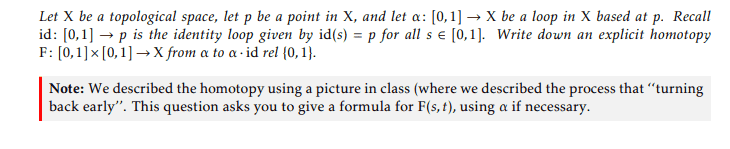# Writing down an explicit homotopy

• I
Mikaelochi
TL;DR Summary
I've never written down an explicit homotopy before which is part of the reason I'm so lost on this problem.I understand that a homotopy is a continuous deformation. The only thing I really remember is something in my notes like this: F(s, 0) = a(s) for 0≤s≤1 and F(s, 1) = a * Id(s). Basically I have to construct some sort of piece-wise function such that I go something like two loops half of the time and stay in place at one point the other half of the time. Anyway, any help is greatly appreciated.

Gold Member
Summary:: I've never written down an explicit homotopy before which is part of the reason I'm so lost on this problem.

View attachment 294278
I understand that a homotopy is a continuous deformation. The only thing I really remember is something in my notes like this: F(s, 0) = a(s) for 0≤s≤1 and F(s, 1) = a * Id(s). Basically I have to construct some sort of piece-wise function such that I go something like two loops half of the time and stay in place at one point the other half of the time. Anyway, any help is greatly appreciated.
I suspect that if you review the definition of multiplication of loops a homotopy will become obvious.

To be entirely rigorous you might like to prove that your homotopy is continuous.

Mikaelochi
I suspect that if you review the definition of multiplication of loops a homotopy will become obvious.

To be entirely rigorous you might like to prove that your homotopy is continuous.
Do you know a good source on that?

Gold Member
@Mikaelochi

It seems that these exercises are designed to get one used to the idea that homotopy classes of loops form a group. For this one needs a loop multiplication that is homotopy invariant. That is: a.b is homotopic to c.d whenever a is homotopic to c and b is homotopic to d. One thinks of a homotopy as a one parameter family of loops that continuously deforms one loop into the other.

Your problem was one of the steps in showing that multiplication forms a group. It shows that multiplication on the right by the constant loop is a right identity.

You also need to show that the constant loop is a left identity and then prove the existence of inverses and the associative law of multiplication. These are all good exercises.

At each base point one gets a different group and one might ask how these groups are related. In mathematics one can compare groups using group homomorphisms. The groups are considered to be in some sense the same if a homomorphism from one to the other has an inverse. This is true for different base points and your exercise on the two base points on the circle is asking you to define an isomorphism.

To get started note that if α is a path connecting the base points p and q and λ is a loop at q then then α followed by λ followed by α in reverse is a loop at p.

BTW: Convince yourself that loop multiplication without homotopies is not a group.

Technicalities:

- By convention loops are defined on the unit interval. So following one loop by another does not give a loop unless it is reparameterized.

- Loops are restricted in your case to have a fixed base point. So a homotopy through loops must keep the base point fixed.

Last edited: